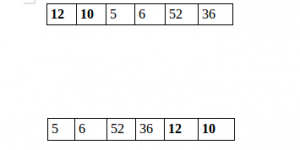# C++ Program to Split the array and add the first part to the end

There is a given an array and split it from a specified position, and move the first part of array add to the end.Examples:

```Input : arr[] = {12, 10, 5, 6, 52, 36}
k = 2
Output : arr[] = {5, 6, 52, 36, 12, 10}
Explanation : Split from index 2 and first
part {12, 10} add to the end .

Input : arr[] = {3, 1, 2}
k = 1
Output : arr[] = {1, 2, 3}
Explanation : Split from index 1 and first
```

## Recommended: Please try your approach on {IDE} first, before moving on to the solution.

 `// CPP program to split array and move first ` `// part to end. ` `#include ` `using` `namespace` `std; ` ` `  `void` `splitArr(``int` `arr[], ``int` `n, ``int` `k) ` `{ ` `    ``for` `(``int` `i = 0; i < k; i++) { ` ` `  `        ``// Rotate array by 1. ` `        ``int` `x = arr; ` `        ``for` `(``int` `j = 0; j < n - 1; ++j) ` `            ``arr[j] = arr[j + 1]; ` `        ``arr[n - 1] = x; ` `    ``} ` `} ` ` `  `// Driver code ` `int` `main() ` `{ ` `    ``int` `arr[] = { 12, 10, 5, 6, 52, 36 }; ` `    ``int` `n = ``sizeof``(arr) / ``sizeof``(arr); ` `    ``int` `position = 2; ` ` `  `    ``splitArr(arr, 6, position); ` ` `  `    ``for` `(``int` `i = 0; i < n; ++i) ` `        ``printf``(``"%d "``, arr[i]); ` ` `  `    ``return` `0; ` `} `

Output:

```5 6 52 36 12 10
```

Please refer complete article on Split the array and add the first part to the end for more details!

My Personal Notes arrow_drop_up

Article Tags :

Be the First to upvote.

Please write to us at contribute@geeksforgeeks.org to report any issue with the above content.